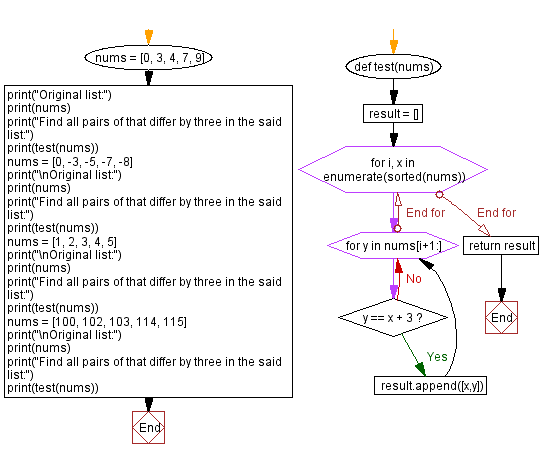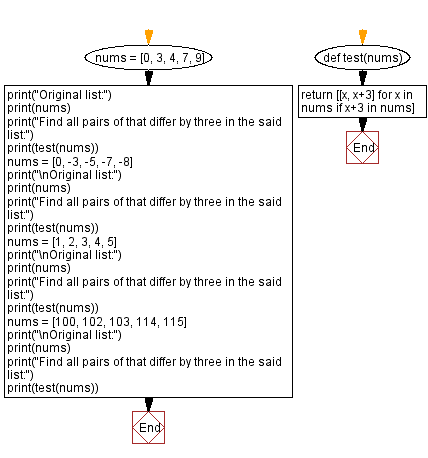﻿ Python - Find all pairs of that differ by three in a list# Python Exercises: Find all pairs of that differ by three in a list

## Python List: Exercise - 281 with Solution

Write a Python program that takes a list of integers and finds all pairs of integers that differ by three. Return all pairs of integers in a list.

Sample Data:
([0, 3, 4, 7, 9]) -> [[0, 3], [4, 7]]
[0, -3, -5, -7, -8] -> [[-3, 0], [-8, -5]]
([1, 2, 3, 4, 5]) -> [[1, 4], [2, 5]]
([100, 102, 103, 114, 115]) -> [[100, 103]]

Sample Solution-1:

Python Code:

``````def test(nums):
result = []
for i, x in enumerate(sorted(nums)):
for y in nums[i+1:]:
if y == x + 3:
result.append([x,y])
return result

nums = [0, 3, 4, 7, 9]
print("Original list:")
print(nums)
print("Find all pairs of that differ by three in the said list:")
print(test(nums))
nums = [0, -3, -5, -7, -8]
print("\nOriginal list:")
print(nums)
print("Find all pairs of that differ by three in the said list:")
print(test(nums))
nums = [1, 2, 3, 4, 5]
print("\nOriginal list:")
print(nums)
print("Find all pairs of that differ by three in the said list:")
print(test(nums))
nums = [100, 102, 103, 114, 115]
print("\nOriginal list:")
print(nums)
print("Find all pairs of that differ by three in the said list:")
print(test(nums))
```
```

Sample Output:

```Original list:
[0, 3, 4, 7, 9]
Find all pairs of that differ by three in the said list:
[[0, 3], [4, 7]]

Original list:
[0, -3, -5, -7, -8]
Find all pairs of that differ by three in the said list:
[[-8, -5]]

Original list:
[1, 2, 3, 4, 5]
Find all pairs of that differ by three in the said list:
[[1, 4], [2, 5]]

Original list:
[100, 102, 103, 114, 115]
Find all pairs of that differ by three in the said list:
[[100, 103]]
```

Flowchart:## Visualize Python code execution:

The following tool visualize what the computer is doing step-by-step as it executes the said program:

Sample Solution-2:

Python Code:

``````def test(nums):
return [[x, x+3] for x in nums if x+3 in nums]

nums = [0, 3, 4, 7, 9]
print("Original list:")
print(nums)
print("Find all pairs of that differ by three in the said list:")
print(test(nums))
nums = [0, -3, -5, -7, -8]
print("\nOriginal list:")
print(nums)
print("Find all pairs of that differ by three in the said list:")
print(test(nums))
nums = [1, 2, 3, 4, 5]
print("\nOriginal list:")
print(nums)
print("Find all pairs of that differ by three in the said list:")
print(test(nums))
nums = [100, 102, 103, 114, 115]
print("\nOriginal list:")
print(nums)
print("Find all pairs of that differ by three in the said list:")
print(test(nums))
```
```

Sample Output:

```Original list:
[0, 3, 4, 7, 9]
Find all pairs of that differ by three in the said list:
[[0, 3], [4, 7]]

Original list:
[0, -3, -5, -7, -8]
Find all pairs of that differ by three in the said list:
[[-3, 0], [-8, -5]]

Original list:
[1, 2, 3, 4, 5]
Find all pairs of that differ by three in the said list:
[[1, 4], [2, 5]]

Original list:
[100, 102, 103, 114, 115]
Find all pairs of that differ by three in the said list:
[[100, 103]]
```

Flowchart:## Visualize Python code execution:

The following tool visualize what the computer is doing step-by-step as it executes the said program:

Python Code Editor:

Have another way to solve this solution? Contribute your code (and comments) through Disqus.

Previous Python Exercise: Extract the first n number of vowels from a string.
Next Python Exercise: Python Dictionary Exercise Home.

What is the difficulty level of this exercise?

Test your Programming skills with w3resource's quiz.

﻿

## Python: Tips of the Day

Getting sorted iterable (can sort by "compare" function):

```>>> a = [1, 2, -3]
>>> sorted(a)
[-3, 1, 2]

>>> sorted(a,key=abs)
[1, 2, -3]
```